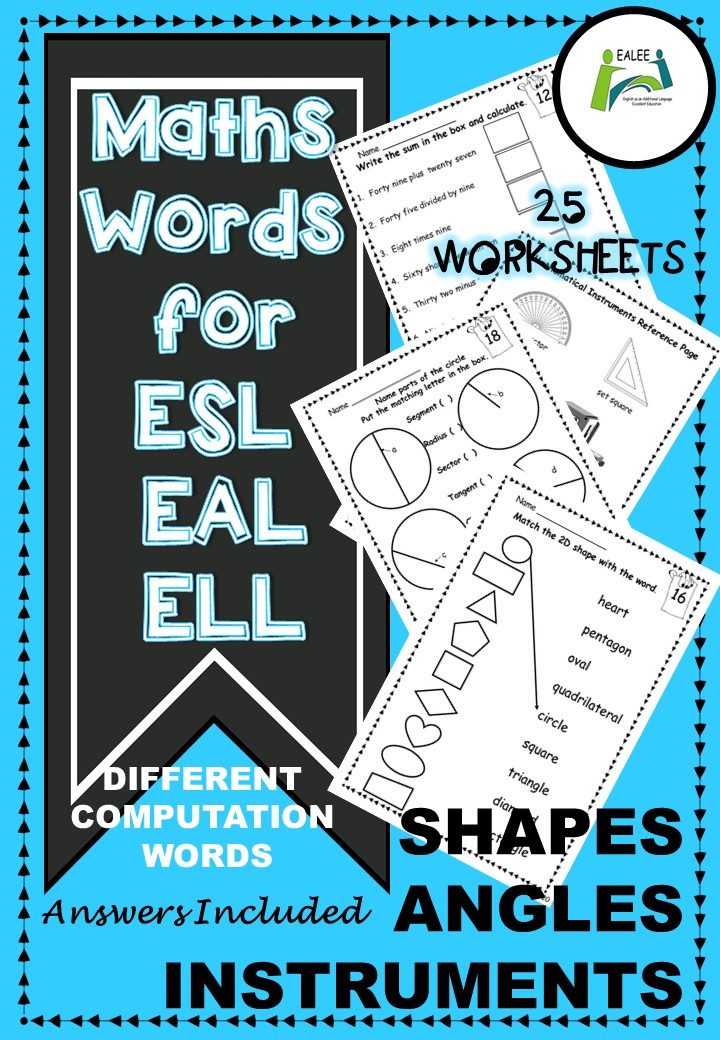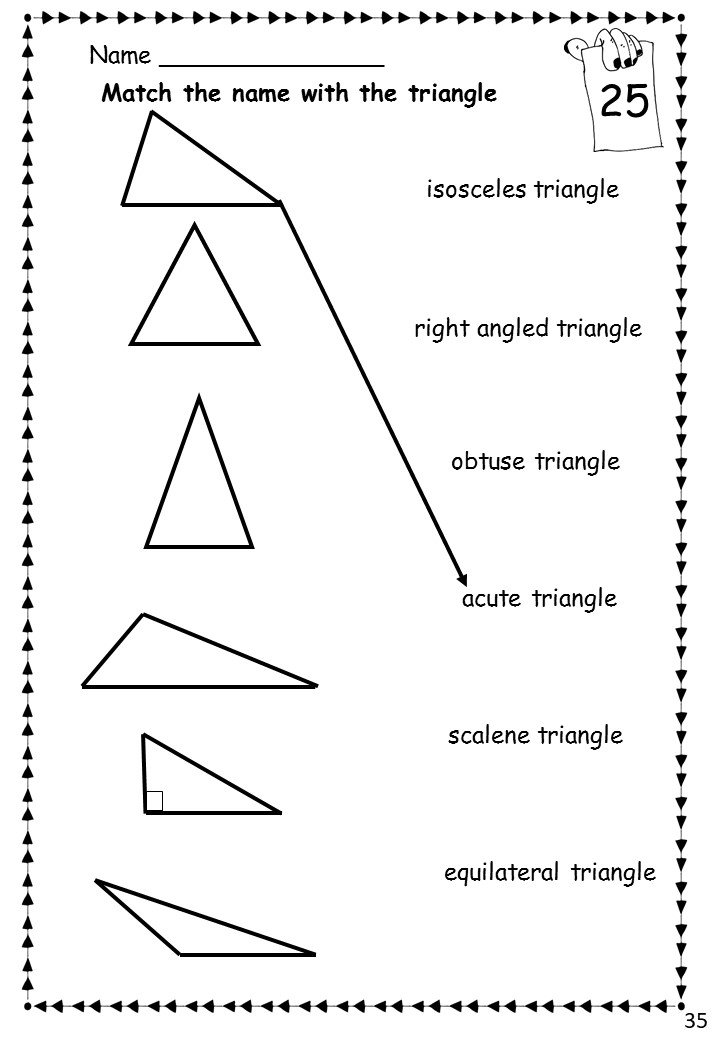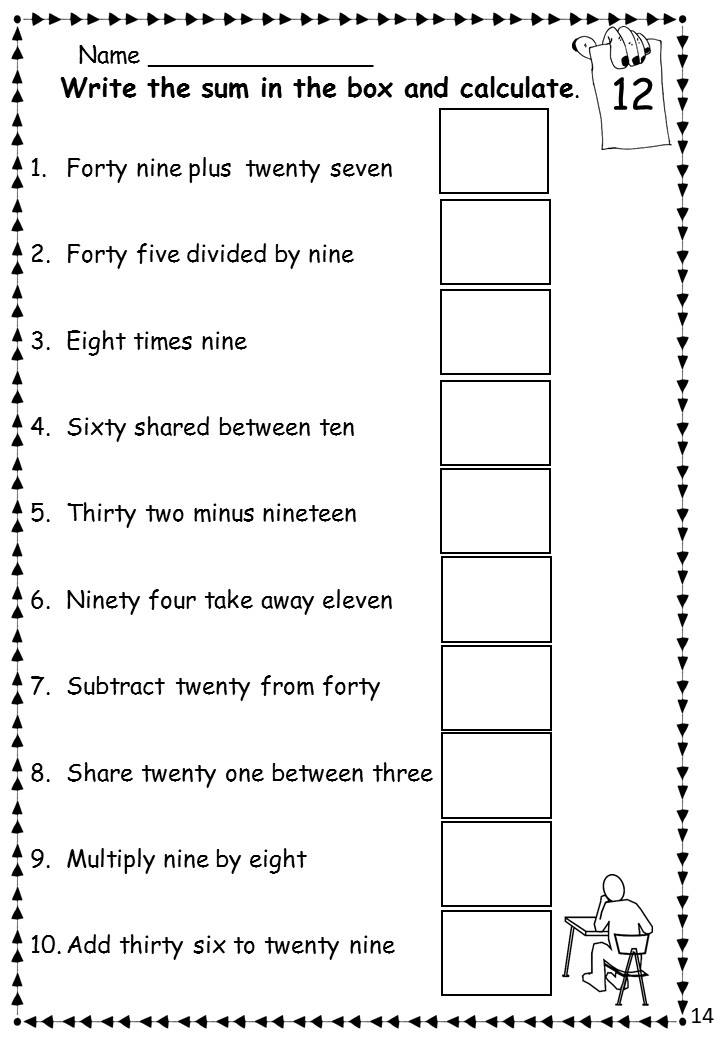##### Math / Maths words for ESL / EAL / ELL
Posted by EALEE - 12 May. 2018 -

https://www.teacherspayteachers.com/Product/Math-Maths-words-for-ESL-EAL-ELL-3809757

This resource is very helpful to newly arrived students who are at the early stage of acquiring English and also useful for native English speakers who are not yet secure with the comprehension of some mathematical terms. Students who are new to English often have difficulty in completing mathematical problems because of lack of English vocabulary, so this resource will be of considerable value to them.     Maths Words for ESL / EAL / ELL provides opportunities for students to develop English vocabulary related to mathematics. By using the reference pages, students are able to successfully complete the 25 worksheets while learning and consolidating new English vocabulary. The 12 reference pages could also be made into booklets and be kept by students for future use.     The 3 contents pages and samples of the resource can be seen in the TpT preview.

THE VOCABULARY

cardinal and ordinal numbers, numbers in words, mathematical signs and related words add, plus, find the sum of, take away, subtract, minus, find the difference, multiply, times, divide, share, even numbers, odd numbers, whole numbers, fractions, numerator, denominator, square, triangle, circle, rectangle, oval, pentagon, quadrilateral, heart, diamond, diameter, radius, circumference, centre, chord, secant, tangent, point of tangency, minor arc, major arc, minor segment, major segment, minor sector, major sector, tangent circles, concentric circles, congruent circles, 2D shapes, 3D shapes, edge, face, vertex, vertices, cone, cube, triangular prism, hemisphere, square based pyramid, cuboid, sphere, triangular based pyramid, cylinder, protractor, set square, compasses, tape measure, dividers, ruler, calculator, right angle, acute angle, obtuse angle, straight angle, reflex angle, a full rotation, acute triangle, equilateral triangle, isosceles triangle, scalene triangle, right angled triangle, obtuse triangle, horizontal line, vertical line, parallel lines, trapezium, perpendicular lines, once, one time, twice, two times, thrice, three times, quadruple, numerical digit

Beginner, Pre-intermediate,
Primary school (ages 5-10), Middle school (ages 11-14), High school (ages 15-18), Adult (18+),
General English, TOEFL,
Printable worksheet,
1.2.3.